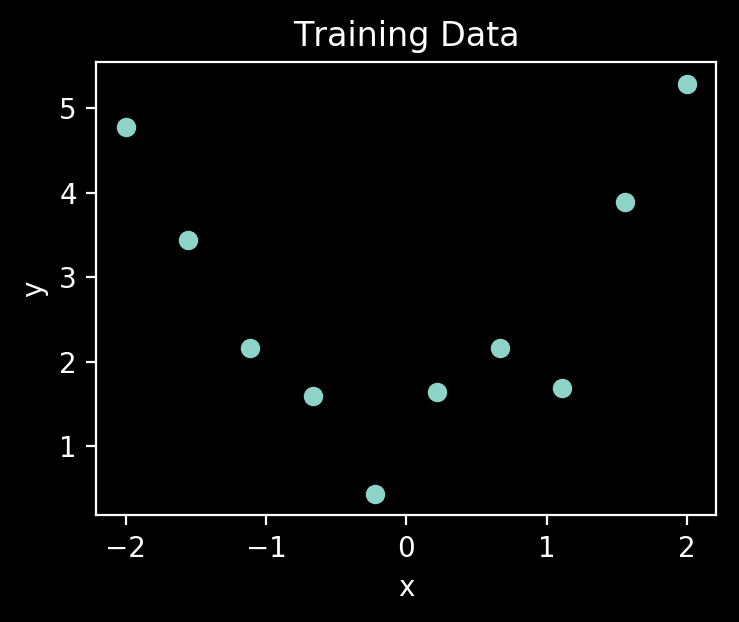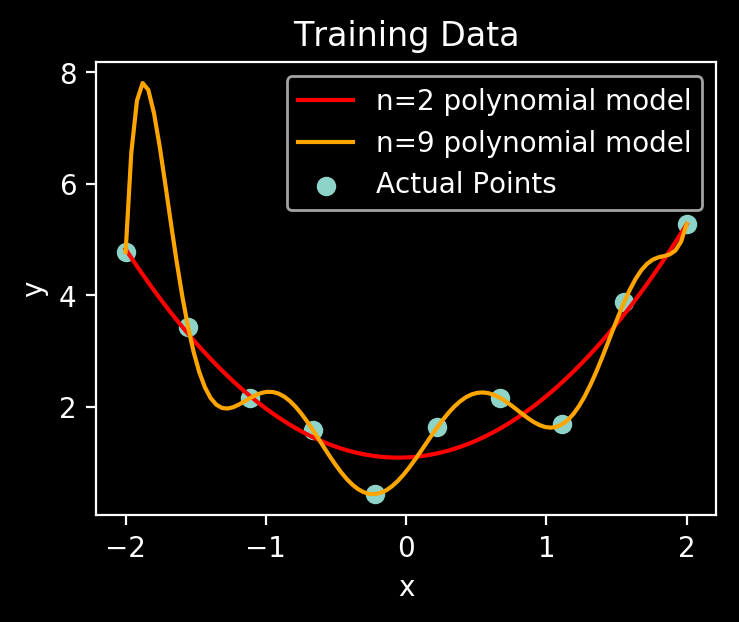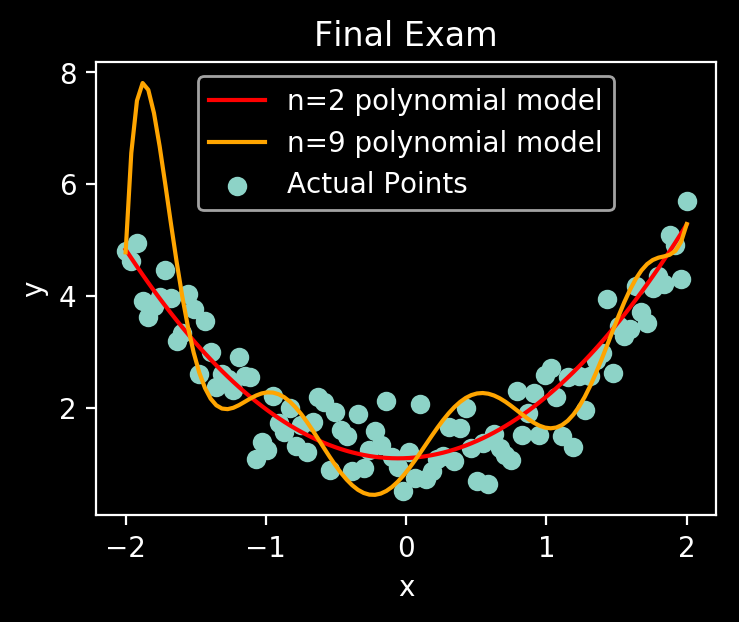# Stats and Bonuses

Jim's blog on data and everything else | 🔮💰👑🤔💻📷🍁🌌🔬🏹

# Machine Learning: Overfitting and k-fold Cross Validation

## Why do we need Cross Validation?¶

From the previous post, we have learned that a pitfall of learning is:

Overfitting on the training data with a powerful model: you memorise the textbook answers but don't know how to do them. You see a similar problem with different numbers on the exam, but don't know what to do

Let's first see an example of overfitting.

# Overfitting Example¶

Consider a 2D plot (10 points) with $y$ vs. $x$.

• Somebody made this plot using a polynomial $y=f(x)=\beta_n x^n + \beta_{n-1} x^{n-1}+ ... + \beta_1 x^1 + \beta_0 x^0 + \epsilon$
• $\epsilon$ is Gaussian noise (different for each data point)
• This person also made a second plot (100 points final exam) using the same function, and gave us 100 $x$-coordinates but not the $y$-coordinates
• We don't know what $n$ is, but we are told $f$ is a polynomial
• We are free to use whatever polynomial we like to approximate $f$

For this final exam, we get a reward of \$100 - (total absolute error of our$y$points vs. the true$y$points), so it is in our best interest to do well. In : import numpy as np # numpy does numbers import matplotlib.pyplot as plt import matplotlib as mpl mpl.style.use('dark_background') # this is the true data-generating function # n=2 in this case def f(x, noise): return(x**2 + 1 + noise) # somebody made the first plot with the above polynomial x_train = np.linspace(-2, 2, 10) noise_train = np.random.normal(0, 0.5, size=10) y_train = f(x_train, noise_train) # plot the training data plt.scatter(x_train, y_train) plt.xlabel('x') plt.ylabel('y') plt.title('Training Data') fig = plt.gcf() fig.set_dpi(200) fig.set_size_inches(4,3, forward=True) plt.show()It's a nice parabola with some noise! ### We now pick a model from the set of all polynomials¶ • If we look at the code above, we know the correct answer is using$n=2$• We will find that higher order polynomials (let's try$n=9$) can fit the data better In : # our two polynomial models model1 = np.poly1d(np.polyfit(x_train, y_train, deg=2)) model2 = np.poly1d(np.polyfit(x_train, y_train, deg=9)) plot_x = np.linspace(-2, 2, 100) # so we can plot a curve plt.scatter(x_train, y_train, label='Actual Points') plt.plot(plot_x, model1(plot_x), label='n=2 polynomial model', color='red') plt.plot(plot_x, model2(plot_x), label='n=9 polynomial model', color='orange') plt.xlabel('x') plt.ylabel('y') plt.title('Training Data') plt.legend() fig = plt.gcf() fig.set_dpi(200) fig.set_size_inches(4,3, forward=True) plt.show()### Which model will perform better on the final exam?¶ If our principle is to select the model that best fits the data, which sounds pretty reasonable, then we will pick the polynomial with$n=9$to use on the final exam. Let's see what happens. In : # final exam---- x_test = np.linspace(-2, 2, 100) # final exam questions noise_test = np.random.normal(0, 0.5, size=100) # answers to the exam, according to the two models y1 = model1(x_test) y2 = model2(x_test) y_test = f(x_test, noise_test) # final exam answer key # the penalty from$100
y1_error = np.sum(np.abs(y_test-y1))
y2_error = np.sum(np.abs(y_test-y2))
print("$$Rewarded----") print("n=2 model:", 100-y1_error) print("n=9 model:", 100-y2_error) plt.scatter(x_test, y_test, label='Actual Points') plt.plot(plot_x, model1(plot_x), label='n=2 polynomial model', color='red') plt.plot(plot_x, model2(plot_x), label='n=9 polynomial model', color='orange') plt.xlabel('x') plt.ylabel('y') plt.title('Final Exam') plt.legend() fig = plt.gcf() fig.set_dpi(200) fig.set_size_inches(4,3, forward=True) plt.show()  $$ Rewarded----
n=2 model: 61.0408020158
n=9 model: 22.5360979023### That orange line is what we call overfitting. Avoid training complex models when data is scarce.¶

• Question: But how will we know we are overfitting before we take the final exam, when it is too late?

# 80:20 split cross validation¶Why are we doing this?

• We can always test on the 20% validation data to check how sober we are
• If we perform poorly on the 20% validation, we will not do well in the final exam
• This is smart, since we won't have (as many) delusions of grandeur

### The problem with 80:20 split¶

If you do the training, validation, tuning cycle too many times, the model will be overfitted to the validation set. How did this come to be?

• You perceive an "improvement" on the validation set test score as an improvement to your model overall
• Obviously, you change your model parameters until you see an improvement in the validation set
• You will gradually do better and better on the validation, partially because you artificially selected models that can spit out good validation numbers by chance
• Eventually your model will reach its useful limits, but you can still tune the model parameters until you get close to memorising the evaluation set. The more complex your model is, the easier it is to do this

# k-fold Cross Validation¶

Idea: If we are not overfitting, then:

• If we can do well on one of the 20% blocks, then if we switch up which data the block points to, we should do equally wellHere, we have $k=5$. The steps to using this:

1. Train on the first training split, then test on the first validation split
2. Clean up the model (reset the model weights)
3. Do the above for splits 2-5
4. Average the scores between validation splits 1-5

Problem: we can still overfit if we try hard enough, even though it is much less likely. We will have to overfit on each of the $k$ validation splits independently in order to overfit.

# Solution: Stratified k-fold CV¶

For validation, why use $\frac{1}{k}$ continuous chunks? We can use random $\frac{1}{k}$ subsamples

Example: we have 10 data points indexed by [1,2,3,4,5,6,7,8,9,10]. Stratified 5-fold CV validation splits:

• [5,3]
• [7,4]
• [1,10]
• [8,6]
• [2,9]

At first glance, this is not very different from the simple 5-fold CV split. Indeed, if we use this split, we are equally likely to overfit as using the [1,2], [3,4], ..., [9,10] split.

### So we randomise the subsamples every time we adjust the hyperparameters of the model. Then it will be very hard to overfit on our validation set. Our validation set isn't even the same thing every time!¶

Example: First time we tune our hyperparameters, the splits look like this:

• [5,3]
• [7,4]
• [1,10]
• [8,6]
• [2,9]

Second time, they look like this:

• [6,1]
• [7,9]
• [3,10]
• [2,5]
• [8,4]

... and so on

Price to pay for using cross validation

• Training models takes time. Compared to the 80:20 split, 5-fold CV takes 5x as long
• $k$-fold CV takes $k$ times as long as the normal split

CV is worth the time.